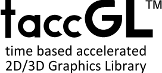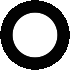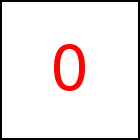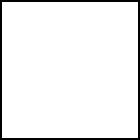# Simple Rotation - taccGL™ Tutorial

## Rotation around the Center of an Element

 taccgl.actor("testimg") .rotateMiddle(0,0,1).start(); RUN taccgl.actor("testimg") .rotateMiddle(0,0,1) .rotatePart(0,Math.PI/2) .start(); RUN

rotateMiddle rotates an element around its mid-point. rotatePart allows you to restrict the rotation from a selected angle to another selected angle. In the second example the starting angle is 0 and the final angle is 90 degrees. The angle needs to be given in radians so PI/2 was specified.

Parameter of rotateMiddle is the rotation axis. (0,0,1) specifies the Z-axis which means a rotation in the X-Y-plane, i.e. in 2D on the screen. The following examples show other axis

 taccgl.actor("testimg") .rotateMiddle(0,1,0) .start(); RUN taccgl.actor("testimg") .rotateMiddle(0,1,0) .rotatePart(0,Math.PI/2) .start(); RUN taccgl.actor("testimg") .rotateMiddle(1,0,0) .start(); RUN taccgl.actor("testimg") .rotateMiddle(1,0,0) .rotatePart(0,Math.PI/2) .start(); RUN

Note that the axis must be a normalized vector, i.e. a vector of length 1.

 taccgl.actor("Layout") .rotateMiddle(1,1,0).start(); RUN taccgl.actor("Layout") .rotateMiddle(1/Math.sqrt(2),1/Math.sqrt(2),0) .start(); RUN

So instead of passing (1,1,0) as axis, the coordinates must be divided by the length of the vector to normalize. In this case the length of the vector is sqrt(1*1+1*1)=sqrt(2).

## Specifying the Rotation Center

With rotate you can rotate around a given point and axis:

 taccgl.actor("Layout") .rotate(100,100,0,0,0,1) .start(); RUN taccgl.actor("Layout") .rotate(1000,100,0,0,0,1) .rotatePart(0,Math.PI/2).start(); RUN taccgl.actor("Layout") .rotate(100,100,0,0,1,0) .start(); RUN taccgl.actor("Layout") .rotate(100,100,0,0,1,0) .rotatePart(0,Math.PI/2).start(); RUN taccgl.actor("Layout") .rotate(100,100,0,1,0,0) .start(); RUN taccgl.actor("Layout") .rotate(100,100,0,1,0,0) .rotatePart(0,Math.PI/2).start(); RUN

WebGL™ is a trademark of the Khronos Group Inc.

Next Page:Accelerated Motion
Previous Page: Basic Animations

# Basic Animations

taccgl™ - HTML5 Canvas Library